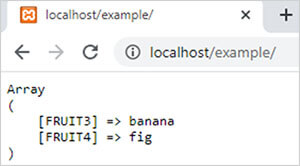PHP array_udiff_uassoc() function || PHP Arrays Functions# array_udiff_uassoc() function in php

1884

PHP array_udiff_uassoc() function is used to return the difference of two or more arrays by comparing the keys and values of arrays with the help of a user-defined function or the callback function.

It is an inbuilt function of PHP.

Syntax:

array_udiff_uassoc(\$array1,\$array2,........,\$arrayN,key_function,value_function);

here,

• \$array1 is a PHP array which unique values is returned by this function.
• \$array2 and \$arrayN are php arrays which values and keys compared to \$array1.
• key_function is a user-defined function which used to compare the keys of arrays. It is mandatory.
• value_function is also a user-defined function which used to compare the values of arrays. It is also mandatory.

Note: The basic difference between array_udiff_assoc() and array_udiff_uassoc() is array_udiff_uassoc() function contains two user defined comparison function one for keys and other for values.

Example 1:

```<?php
function key_function(\$x,\$y)
{
if (\$x===\$y)
{
return 0;
}
return (\$x>\$y)?1:-1;
}
function value_function(\$x,\$y)
{
if (\$x===\$y)
{
return 0;
}
return (\$x>\$y)?1:-1;
}
\$arr1=array("FRUIT1"=>"apple","FRUIT2"=>"guava","FRUIT3"=>"banana","FRUIT4"=>"fig");
\$arr2=array("FRUIT1"=>"apple","FRUIT2"=>"guava","FRUIT3"=>"apple","FRUIT4"=>"guava");
\$result=array_udiff_uassoc(\$arr1,\$arr2,"key_function","value_function");
echo "<pre>";
print_r(\$result);
?>```

Output:Share:## Big Hosting Days

Upto 75@ OFF on Web Hosting

## Get a .COM for just \$6.98

Secure Domain for a Mini Price